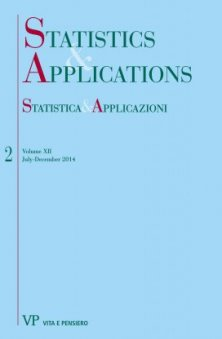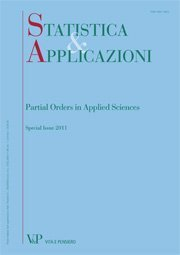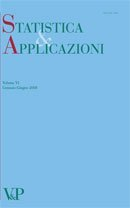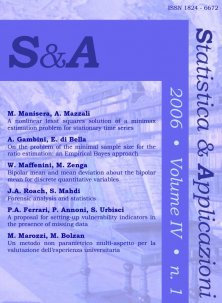Libri di Marica Manisera - libri Statistica & Applicazioni

# Marica Manisera

### Author's titles

Nonlinear CUB models: the R code digitalYear: 2015
Nonlinear CUB models have been recently introduced in the literature to model ordinal data taking into account the unequal spacing among response categories. Nonlinear CUB models can be effectively used in a variety of fields, typically when human perceptions and attitudes are measured by questionnaires with questions having ordered response categories...
Interpreting clusters and their bipolar means: a case study
Free
digitalYear: 2013
When cluster analysis is performed on ranking or rating data, methods requiring quantitative variables commonly used to characterize the obtained groups, such as the cluster profile plots, are not appropriate. Instead, the bipolar mean, originally introduced in the literature in 2005 to deal with such kind of data, can be useful to interpret the resulting clusters, possibly in association with other available information. An application on real data coming from an extensive survey carried out in 2011 in the Italian McDonald’s restaurants is presented. A selection of ranking data, regarding some features of products and service, was analysed by a hierarchical cluster algorithm. In order to emphasize the concordance between the most important ranks, a weighted rank correlation coefficient was employed to measure the dissimilarity between respondents. Five groups were finally obtained, which show interesting differences on the given rankings.
Models for categorical data: a comparison between the Rasch model and Nonlinear Principal Component Analysis
Free
digitalYear: 2007
The paper compares two models to construct measures from the responses on a set of categorical variables, the Rasch Model and the Nonlinear (Categorical) Principal Component Analysis, and can be considered as a part of the literature about the choice between stochastic and algorithmic models. The aim is to discuss the Rasch Model and Nonlinear PCA differences and similarities, emphasizing the information that can be drawn from the data, and to compare the resulting measures.
A Nonlinear Least Squares Solution of a Minimax Estimation Problem for Stationary Time Series digitalYear: 2006
The parameter estimation problem for the ARMA models is considered under a minimax approach: when the objective is to minimize the largest deviations in time series, it is useful to define a generalized version of a minimax estimator, minimizing the sum of the r-th powers of the k